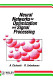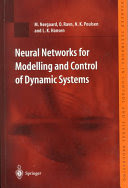Intelligent Control Systems

Course Description

Classification of artificial neural networks. Neural network (NN) structures suitable for system modeling and control. NN learning algorithms. Application of NN in identification and modeling of complex systems. Selection of suitable NN structures and their validation. NN process model-based control structures. Application of NN for improvement of nonlinear control system performance. Concept of current NN linearization and its application to linear controller synthesis. Application of NN for compensation of system ambiguities. Basic of evolutionary and genetic optimization algorithms.

General Competencies

Knowledge about complex neural network controller design. Knowledge about different learning and automated setting methods for parameters tuning of intelligent control algorithms.

Learning Outcomes

1. classify artificial neural networks
2. apply nonrecursive neural network learning algorithms
3. apply recursive neural network learning algorithms
4. identify nonlinear dynamical systems via static neural networks
5. design a neural controller for control of nonlinear dynamical systems
6. apply genetic algorithms in optimization

Forms of Teaching

Lectures

Lectures are organized in two thematic cycles (neural networks and genetic algorithms).

Exams

Seminars. Direct conversation with students in the classroom.

Consultations

One hour weekly.

Seminars

Neural network design for identification and/or control of nonlinear dynamical system.

Acquisition of Skills

Use of Matlab Neural Networks Toolbox.

Continuous Assessment Exam
Type Threshold Percent of Grade Threshold Percent of Grade
Seminar/Project 0 % 35 % 0 % 35 %
Mid Term Exam: Written 0 % 20 % 0 %
Final Exam: Written 0 % 20 %
Final Exam: Oral 25 %
Exam: Written 0 % 40 %
Exam: Oral 25 %
Comment:

The oral exam share is ±25%. Seminars are obligatory.

Week by Week Schedule

1. Introduction to artificial neural networks. Classification of artificial neural networks.
2. Static neural networks. MultiLayer Perceptron neural networks. Radial Basis Function (RBF) neural networks.
3. Dynamic neural networks. Neural networks training.
4. Non-recursive algorithms for neural networks training. Gradient based algorithms.
5. Newton algorithms. Quasi-Newton algorithms.
6. Recursive learning algorithms.
7. Midterm exam
8. Neural network (NN) structures suitable for system modeling and control. NN learning algorithms.
9. Application of NN in identification and modeling of complex systems. Selection of suitable NN structures and their validation.
10. NN process model-based control structures. Application of NN for improvement of nonlinear control system performance.
11. Concept of instantaneous NN linearization and its application to linear controller synthesis.
12. Application of NN for compensation of system uncertainties.
13. Genetic algorithms (GA). Selection of population entities. Crossover operators. Mutation operators. Elitism.
14. Selection of penalty functions. Applications of GA.
15. Final exam

Study Programmes

Control Engineering and Automation (profile)
Recommended elective courses (3. semester)
Electrical Engineering Systems and Technologies (profile)
Recommended elective courses (3. semester)

LiteratureA. Cichocki, R. Unbehauen (1993.), Neural Networks for Optimization and Signal Processing, John Wiley & SonsM. Nørgaard, O. Ravn, N. K. Poulsen, L. K. Hansen (2000.), Neural Networks for Modelling and Control of Dynamic Systems, Springer-Verlag, London
Ivan Petrović, Mato Baotić, Nedjeljko Perić (2015.), Inteligentni sustavi upravljanja: neuronske mreže i genetički algoritmi, Sveučilište u Zagrebu Fakultet elektrotehnike i računarstva

General

ID 34381
Winter semester
4 ECTS
L1 English Level
L1 e-Learning
30 Lectures
0 Exercises
0 Laboratory exercises
0 Project laboratory